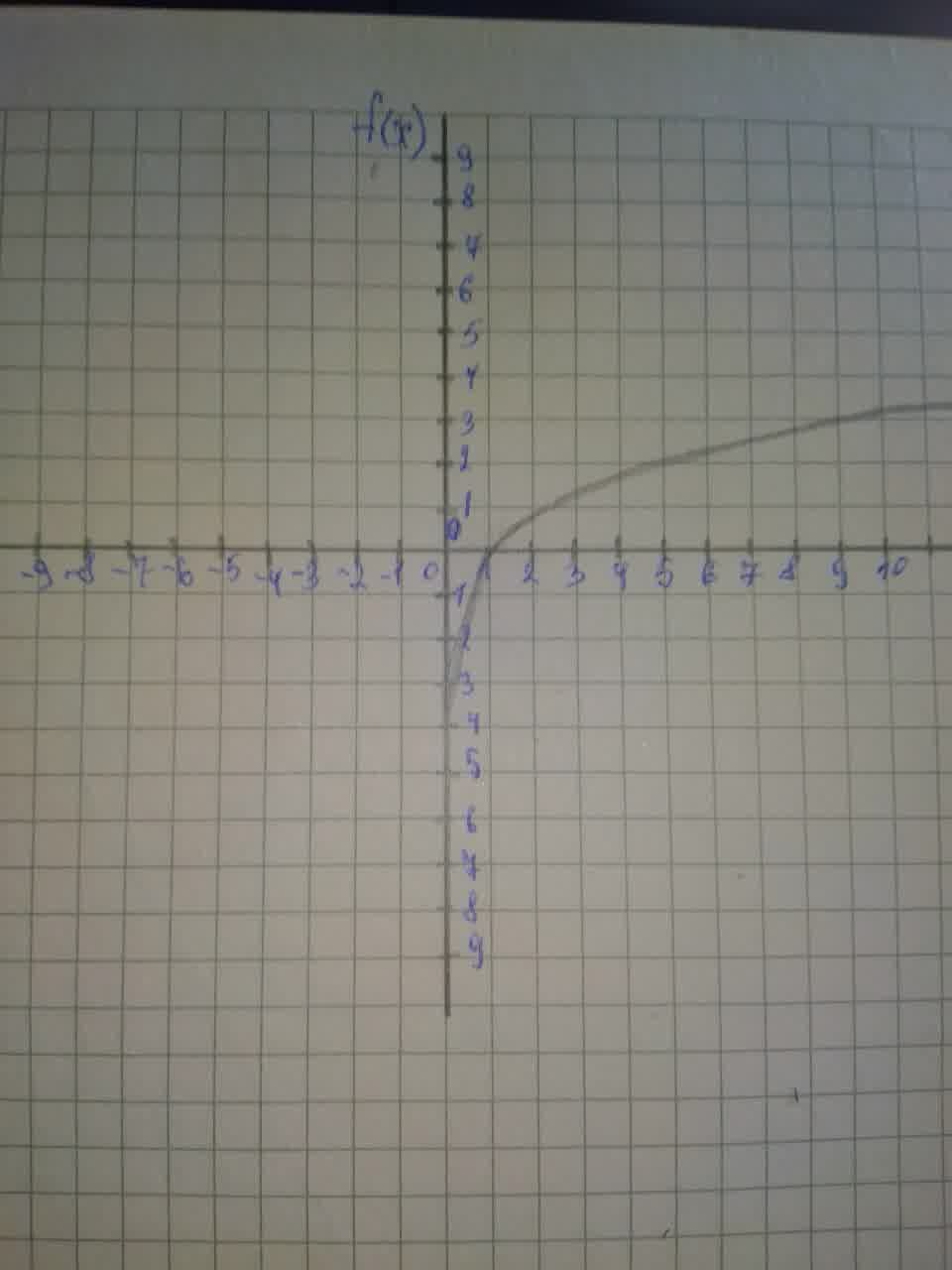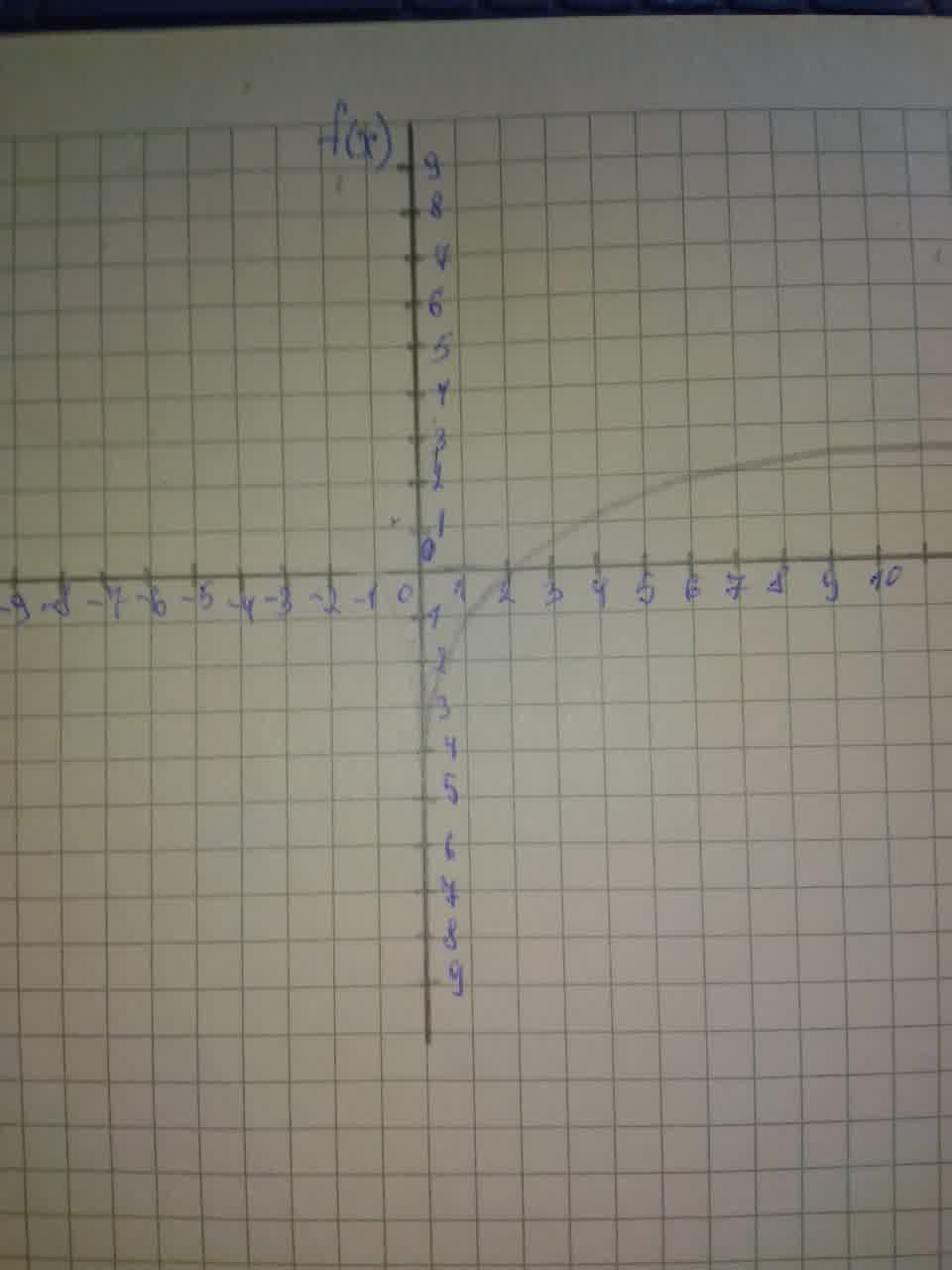Begin by graphing f(x)= \log_{2}x and then use transformations of this graph to graph the given function: h(x)=-1+ \log_{2}xtricotasu 2021-08-05 Answered

Begin by graphing
$$f(x)= \log_{2}x.$$
Then use transformations of this graph to graph the given function. What is the graph's x-intercept? What is the vertical asymptote? Use the graphs to determine each functions domain and range.
$$\displaystyle{h}{\left({x}\right)}=-{1}+{{\log}_{{{2}}}{x}}$$

• Questions are typically answered in as fast as 30 minutes

Solve your problem for the price of one coffee

• Math expert for every subject
• Pay only if we can solve itAniqa O'Neill

Step 1
Graph of $$\displaystyle{f{{\left({x}\right)}}}={{\log}_{{{2}}}{x}}$$Step 2Answer: Graph of $$\displaystyle{h}{\left({x}\right)}=-{1}+{{\log}_{{{2}}}{x}}$$. The graph is translated down 1 unit from the graph f(x).
The vertical asymptote is $$\displaystyle{x}={0}$$. The x-intercept is (2,0. The domain of h(x) is $$\displaystyle{\left\lbrace{x}{\mid}{x}{>}{0}\right\rbrace}$$. The range is all real numbers.